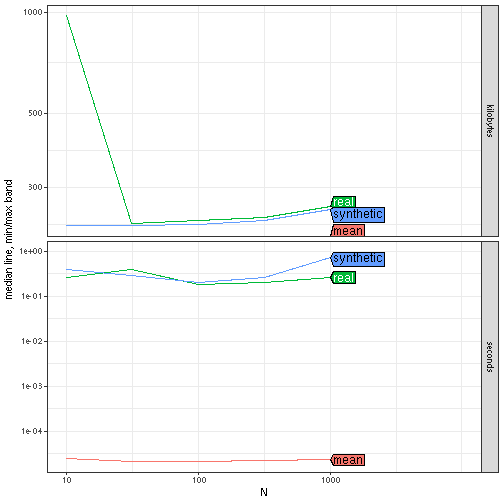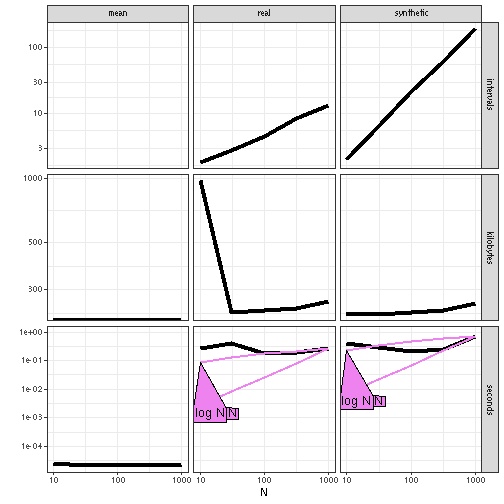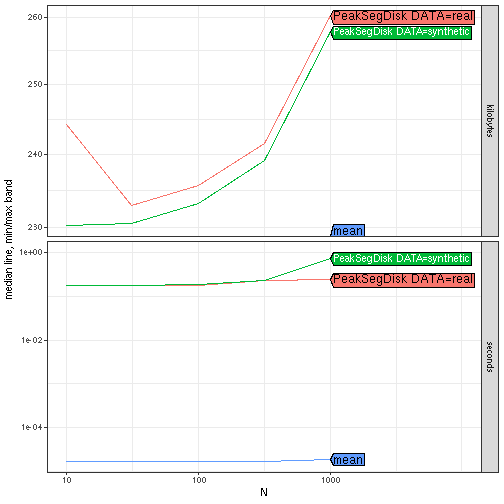# Custom Units

The goal of this vignette is to explain how to estimate asymptotic complexity for custom units (other than the defaults, seconds and kilobytes).

## Dynamic programming algorithms for change-point detection

The time complexity of the Dynamic Programming algorithm implemented in the PeakSegDisk package depends on the number of intervals (candidate changepoints stored). Here we compute the mean number of intervals for real Mono27ac data, and synthetic count data which are always increasing.

``````old.opt <- options(width=100)
data(Mono27ac, package="PeakSegDisk", envir=environment())
library(data.table)
penalty <- 1e6
expr.list <- c(
if(requireNamespace("PeakSegDisk"))atime::atime_grid(
real=PeakSegDisk::PeakSegFPOP_df(real, penalty),
synthetic=PeakSegDisk::PeakSegFPOP_df(synthetic, penalty)),
atime::atime_grid(mean=mean(real\$count)))
atime.list <- atime::atime(
N=as.integer(10^seq(1, 3, by=0.5)),
setup={
real <- Mono27ac\$coverage[1:N]
synthetic <- data.table(real)[, count := 1:.N]
},
expr.list=expr.list,
seconds.limit=Inf,
result=TRUE)
plot(atime.list)
#> Warning: Transformation introduced infinite values in continuous y-axis
#> Transformation introduced infinite values in continuous y-axis
#> Transformation introduced infinite values in continuous y-axis
#> Warning in grid.Call.graphics(C_polygon, x\$x, x\$y, index): semi-transparency is not supported on
#> this device: reported only once per page
``````The plot above shows the timings in both kinds of data. Clearly the algorithm is much faster in real data than in synthetic increasing data. The code below creates a new column for the mean number of intervals computing during the algorithm, then computes the best asymptotic references:

``````atime.list\$measurements[, intervals := sapply(
result, function(L)if(is.numeric(L))NA else L\$loss\$mean.intervals)]
best.list <- atime::references_best(atime.list, more.units="intervals")
plot(best.list)
#> Warning: Transformation introduced infinite values in continuous y-axis
#> Warning: Removed 5 rows containing missing values (`geom_line()`).
#> Warning in grid.Call.graphics(C_polygon, x\$x, x\$y, index): semi-transparency is not supported on
#> this device: reported only once per page
``````Note in the code above the `more.units="intervals"` argument, which says to use the intervals column as an additional unit. The plot above shows plots of all three units as a function of data size. It is clear that there is a substantial difference in the number of intervals stored by the algorithm, between real and synthetic increasing data. From the plot above it is clear that

• the number of intervals grows slowly (log) for real data, and much faster (linear) for synthetic increasing data,
• the memory usage (kilobytes) is grows slowly (log or constant),
• the computation time grows slowly for real data (expected log-linear), and much faster for synthetic increasing data (expected quadratic).

Exercise for the reader: to see the expected asymptotic time complexity in the last plot, re-do the previous analyses, increasing the penalty as well as the max data size N.

``````options(old.opt)
``````

## Doing the same benchmark using `atime_grid`

Note in the original `atime` call above, the only difference between real and synthetic is the data, so the `atime_grid` function could be used to create an expression list in this case. The advantage is that the code below avoids the repetition of the `PeakSegFPOP_df` function call, which would be even more beneficial if there were more than two data sets:

``````(data.grid.exprs <- c(
if(requireNamespace("PeakSegDisk"))atime::atime_grid(
list(DATA=c("real","synthetic")),
PeakSegDisk=PeakSegDisk::PeakSegFPOP_df(data.list[[DATA]], penalty)),
atime::atime_grid(mean=mean(data.list\$real\$count))))
#> \$`PeakSegDisk DATA=real`
#> PeakSegDisk::PeakSegFPOP_df(data.list[["real"]], penalty)
#>
#> \$`PeakSegDisk DATA=synthetic`
#> PeakSegDisk::PeakSegFPOP_df(data.list[["synthetic"]], penalty)
#>
#> \$mean
#> mean(data.list\$real\$count)
data.grid.result <- atime::atime(
N=as.integer(10^seq(1, 3, by=0.5)),
setup={
real <- Mono27ac\$coverage[1:N]
data.list <- list(
real=real,
synthetic=data.table(real)[, count := 1:.N])
},
seconds.limit = Inf,
expr.list=data.grid.exprs)
plot(data.grid.result)
#> Warning: Transformation introduced infinite values in continuous y-axis
#> Transformation introduced infinite values in continuous y-axis
#> Transformation introduced infinite values in continuous y-axis
#> Warning in grid.Call.graphics(C_polygon, x\$x, x\$y, index): semi-transparency is
#> not supported on this device: reported only once per page
``````